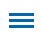Seventh-grade students are asked to "use properties of operations to generate equivalent expressions." While they may be able to solve these equations, they don't often make the connection between abstract algebra formulas and the world outside the classroom.

Use this 13-page Wixie template to provide a real-world context for using algebraic equations as students are given parameters and asked to price jewelry based on the algebraic expression they write.

Editor's Note: This blog is the sixth in a series of posts from former math specialist Scott Loomis. In these posts, he shares more about the real world math templates he has created for Wixie and outlines how you can use and modify them for your own awesome performance tasks. You can read the backstory on his experience and expertise below.

Instructional Concept: Solve real-life and mathematical problems using algebraic expressions and equations.Location: Templates > Math > Algebra

Estimated Completion Time: 25 minutes

Common Core State Standards for Mathematics

CCSS.MATH.CONTENT.7.EE.B.4
Use variables to represent quantities in a real-world or mathematical problem, and construct simple equations and inequalities to solve problems by reasoning about the quantities.